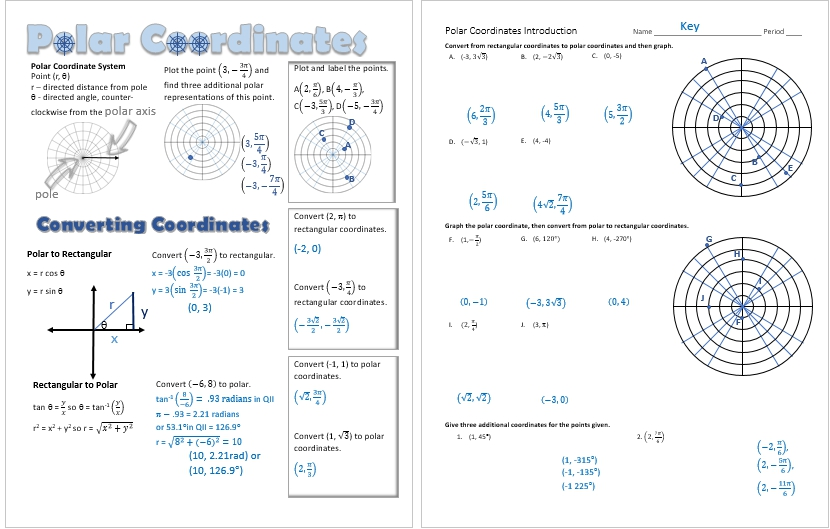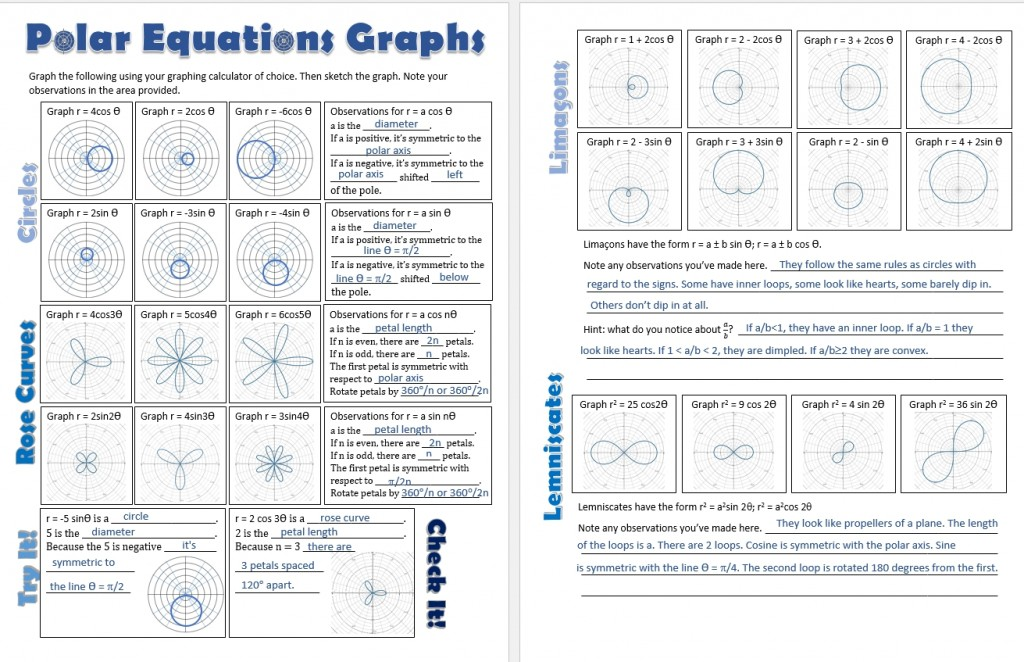Polar Coordinates and Graphs

/, PreCalculus/Polar Coordinates and Graphs

Polar Coordinates and Graphs

I remember being frightened by the polar coordinate system and graphing equations using it when I was in my students’ seats many years ago. With graphing calculators, this doesn’t have to be intimidating.

I started the unit with an introduction to the polar coordinate system and reminded my students about how intimately familiar they are with the unit circle and how closely this resembles it. I passed out the Vizual Notes and we learned how to plot points, name a point in three additional ways, and how to convert between the two systems. Then my students practiced using their new knowledge.Next we discussed how to convert rectangular equations to polar and vice versa.

Then it’s on to an investigation to see how much my students can learn by graphing polar equations using a graphing calculator.By | 2019-02-07T20:50:54+00:00 April 9th, 2017|Graphing Calculator, PreCalculus|0 Comments